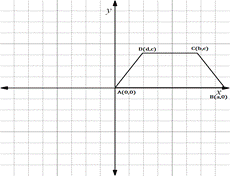Chapter 10.CT, Problem 11CTElementary Geometry For College St...

7th Edition
Alexander + 2 others
ISBN: 9781337614085

Solutions

Chapter
SectionElementary Geometry For College St...

7th Edition
Alexander + 2 others
ISBN: 9781337614085
Textbook Problem

Being as specific as possible, decribe the type of polygon shown in each figure.(a)(b)

To determine

(a)

To describe:

The type of polygon shown in the given figure.

Explanation

The given figure is shown below.

Find the distance RT.

x1,y1=R-a, 0

x2,y2=T0, b

Formula for distance between two points

d=x2-x12+y2-y12

Substituting the x and y co-ordinates

dRT=0--a2+b-02

On solving this,

dRT=a2+b2

To determine

(b)

To describe:

The type of polygon shown in the given figure.

Still sussing out bartleby?

Check out a sample textbook solution.

See a sample solution

The Solution to Your Study Problems

Bartleby provides explanations to thousands of textbook problems written by our experts, many with advanced degrees!

Get Started

Find more solutions based on key concepts